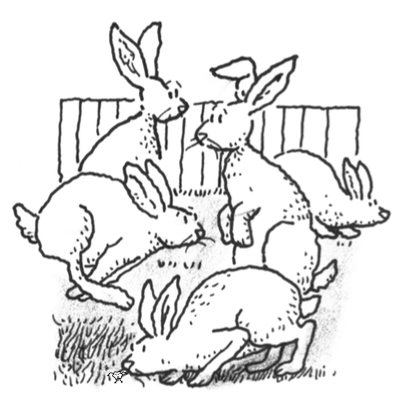### Home > APCALC > Chapter 10 > Lesson 10.1.7 > Problem10-84

10-84.

The rabbits in your neighbor’s backyard are reproducing at a rate proportional to the number present. Today there are $32$ rabbits. Two weeks ago there were only $20$. When will there be $200$ rabbits?

If $P =$ rabbit population, then:

$\frac{dP}{dt}=kP$

Use separation of variables then integrate to obtain:

$P=Ce^{kt}$

Solve for $C$ and $k$:

$32=Ce^{0k}\text{ and }20=Ce^{-2k}$

Solve for $t$:

$200=32e^{-0.5\ln(20/32)t}$